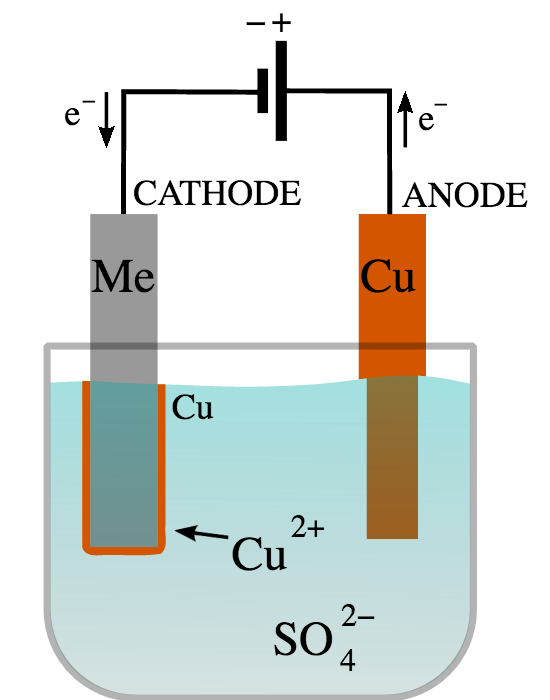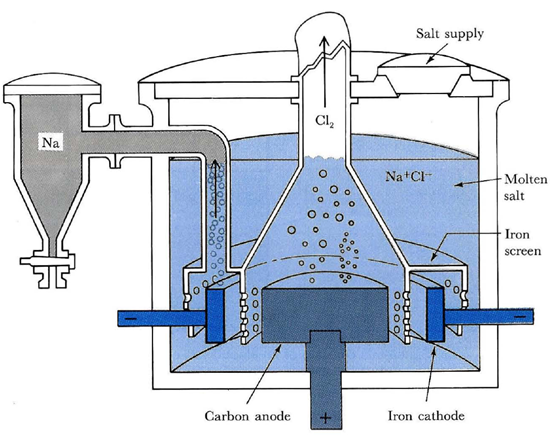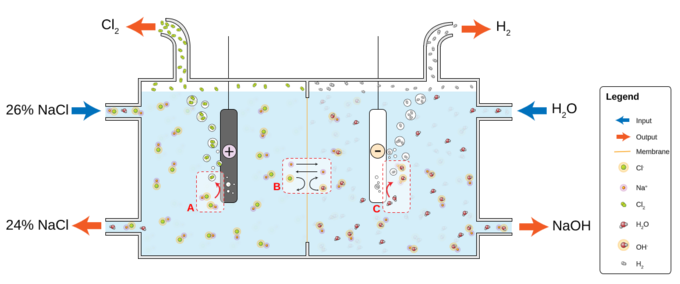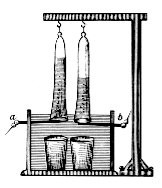## Predicting the Products of Electrolysis

Electrolysis is a way of separating a compound by passing an electric current through it; the products are the compound’s component ions.

### Learning Objectives

Predict the products of an electrolysis reaction

### Key Takeaways

#### Key Points

• The main components of an electrolytic cell are an electrolyte, DC current, and two electrodes.
• The key process of electrolysis is the interchange of atoms and ions by the removal or addition of electrons to the external circuit.
• Oxidation of ions or neutral molecules occurs at the anode, and reduction of ions or neutral molecules occurs at the cathode.

#### Key Terms

• electrolyte: A substance that, in solution or when molten, ionizes and conducts electricity.
• electrolysis: The chemical change produced by passing an electric current through a conducting solution or a molten salt.

### What Is Electrolysis?

In order to predict the products of electrolysis, we first need to understand what electrolysis is and how it works. Electrolysis is a method of separating bonded elements and compounds by passing an electric current through them. It uses a direct electric current (DC) to drive an otherwise non-spontaneous chemical reaction. Electrolysis is very important commercially as a stage in the separation of elements from naturally occurring sources, such as ores, using an electrolytic cell.

The main components required to achieve electrolysis are:

• An electrolyte: a substance containing free ions, which are the carriers of electric current in the electrolyte. If the ions are not mobile, as in a solid salt, then electrolysis cannot occur.
• A direct current (DC) supply: provides the energy necessary to create or discharge the ions in the electrolyte. Electric current is carried by electrons in the external circuit.
• Two electrodes: an electrical conductor that provides the physical interface between the electrical circuit providing the energy and the electrolyte.

### The Interchange of Atoms and Ions

The key process of electrolysis is the interchange of atoms and ions by the removal or addition of electrons to the external circuit. The required products of electrolysis are in a different physical state from the electrolyte and can be removed by some physical processes.

Each electrode attracts ions that are of the opposite charge. Positively charged ions, or cations, move toward the electron-providing cathode, which is negative; negatively charged ions, or anions, move toward the positive anode. You may have noticed that this is the opposite of a galvanic cell, where the anode is negative and the cathode is positive.

At the electrodes, electrons are absorbed or released by the atoms and ions. Those atoms that gain or lose electrons become charged ions that pass into the electrolyte. Those ions that gain or lose electrons to become uncharged atoms separate from the electrolyte. The formation of uncharged atoms from ions is called discharging. The energy required to cause the ions to migrate to the electrodes, and the energy to cause the change in ionic state, is provided by the external source.

### Oxidation and Reduction

Oxidation of ions or neutral molecules occurs at the anode, and reduction of ions or neutral molecules occurs at the cathode. For example, it is possible to oxidize ferrous ions to ferric ions at the anode:

$\text{Fe}^{2+} (\text{aq}) \rightarrow \text{Fe}^{3+} (\text{aq}) + \text{e}^-$

It is also possible to reduce ferricyanide ions to ferrocyanide ions at the cathode:

$\text{Fe}(\text{CN})^{3-}_6 + \text{e}^- \rightarrow \text{Fe}(\text{CN})^{4-}_6$

Neutral molecules can also react at either electrode. Electrolysis reactions involving H+ ions are fairly common in acidic solutions. In alkaline water solutions, reactions involving hydroxide ions (OH) are common. The substances oxidized or reduced can also be the solvent, which is usually water, or the electrodes. It is possible to have electrolysis involving gases.

### Predicting the Products of Electrolysis

Let’s look at how to predict the products. For example, what two ions will CuSO4 break down into? The answer is Cu2+ and SO42-. Let’s look more closely at this reaction.Electrolysis of copper sulfate: Two copper electrodes are placed in a solution of blue copper sulfate and are connected to a source of electrical current. The current is turned on for a period of time.

We take two copper electrodes and place them into a solution of blue copper sulfate (CuSO4) and then turn the current on. We notice that the the initial blue color of the solution remains unchanged, but it appears that copper has been deposited on one of the electrodes but dissolved on the other. This is because Cu2+ ions are attracted to the negatively charged cathode, and since the the cathode is putting out electrons, the Cu2+ becomes reduced to form copper metal, which is deposited on the electrode. The reaction at this electrode is:

$\text{Cu}^{2+} (\text{aq}) + 2\text{e}^- \rightarrow \text{Cu} (\text{s})$

At the positive anode, copper metal is oxidized to form Cu2+ ions. This is why it appears that the copper has dissolved from the electrode. The reaction at this electrode is:

$\text{Cu} (\text{s}) \rightarrow \text{Cu}^{2+} (\text{aq}) + 2\text{e}^-$

We just saw electric current used to split CuSO4 into its component ions. This is all it takes to predict the products of electrolysis; all you have to do is break down a compound into its component ions.

## Electrolysis of Sodium Chloride

Two commonly used methods of electrolysis involve molten sodium chloride and aqueous sodium chloride, which give different products.

### Learning Objectives

Predict the products of electrolysis of sodium chloride under molten and aqueous conditions

### Key Takeaways

#### Key Points

• Sodium metal and chlorine gas can be obtained with the electrolysis of molten sodium chloride.
• Electrolysis of aqueous sodium chloride yields hydrogen and chlorine, with aqueous sodium hydroxide remaining in solution.
• The reason for the difference is that the reduction of Na+ (E° = –2.7 v) is energetically more difficult than the reduction of water (–1.23 v).

#### Key Terms

• anode: The electrode of an electrochemical cell at which oxidation occurs.
• cathode: The electrode of an electrochemical cell at which reduction occurs.

### Electrolysis of NaCl

As we have covered, electrolysis is the passage of a direct electric current through an ionic substance that is either molten or dissolved in a suitable solvent. This results in chemical reactions at the electrodes and the separation of materials. Two commonly used methods of electrolysis involve molten sodium chloride and aqueous sodium chloride. You might think that both methods would give you the same products, but this not the case. Let’s go through each of the methods to understand the different processes.

### Electrolysis of Molten NaCl

If sodium chloride is melted (above 801 °C), two electrodes are inserted into the melt, and an electric current is passed through the molten salt, then chemical reactions take place at the electrodes.Electrolysis cell for molten sodium chloride: A commercial electrolysis cell for the production of metallic sodium and chlorine gas from molten NaCl. Liquid sodium floats to the top of the melt above the cathode and is drained off into a storage tank. Chlorine gas bubbles out of the melt above the anode.

Sodium ions migrate to the cathode, where electrons enter the melt and are reduced to sodium metal:

${\text{Na}}^{+} + {\text{e}}^{-} \rightarrow \text{Na}$

Chloride ions migrate the other way, toward the anode. They give up their electrons to the anode and are oxidized to chlorine gas:

${\text{Cl}}^{-} \rightarrow \frac{1}{2}{\text{Cl}}_{2} + {\text{e}}^{-}$

The overall reaction is the breakdown of sodium chloride into its elements:

$2\text{NaCl} \rightarrow 2\text{Na}(\text{s}) + {\text{Cl}}_{2}(\text{g})$

### Electrolysis of Aqueous NaCl

What happens when we have an aqueous solution of sodium chloride? Well, we can’t forget that we have to factor water into the equation. Since water can be both oxidized and reduced, it competes with the dissolved Na+ and Cl ions. Rather than producing sodium, hydrogen is produced.Electrolysis of aqueous sodium chloride: Electrolysis of aqueous NaCl results in hydrogen and chloride gas. At the anode (A), chloride (Cl-) is oxidized to chlorine. The ion-selective membrane (B) allows the counterion Na+ to freely flow across, but prevents anions such as hydroxide (OH-) and chloride from diffusing across. At the cathode (C), water is reduced to hydroxide and hydrogen gas. The net process is the electrolysis of an aqueous solution of NaCl into industrially useful products sodium hydroxide (NaOH) and chlorine gas.

The reaction at the cathode is:

${\text{H}}_{2}\text{O} (\text{l}) + 2 {\text{e}}^{- } \rightarrow {\text{H}}_{2}(\text{g}) + 2{ \text{OH}}^{- }$

The reaction at the anode is:

${\text{Cl}}^{- } \rightarrow \frac{1}{2} {\text{Cl}}_{2}(\text{g}) +1\text{e}^-$

The overall reaction is as follows:

$\text{NaCl}(\text{aq}) + {\text{H}}_{2}\text{O}(\text{l}) \rightarrow {\text{Na}}^{+}(\text{aq}) + {\text{OH}}^{-}(\text{aq}) + {\text{H}}_{2}(\text{g}) + \frac{1}{2}{\text{Cl}}_{2}(\text{g})$

Reduction of Na+ (E° = –2.7 v) is energetically more difficult than the reduction of water (–1.23 v), so in aqueous solution, the latter will prevail.

Deduce the products of the electrolysis of a molten salt: Electrolysis of a molten salt produces the elements from the salt. So, the electrolysis of WCl4 produces W and Cl2. Metal ions receive electrons at the negative electrode, and the non-metals lose them at the positive electrode.

## Electrolysis of Water

Pure water cannot undergo significant electrolysis without an electrolyte, such as an acid or a base.

### Learning Objectives

Recall the properties of an electrolyte that enable the electrolysis of water

### Key Takeaways

#### Key Points

• Electrolysis of a solution of sulfuric acid or of a salt, such as NaNO3, results in the decomposition of water at both electrodes.
• Hydrogen will appear at the cathode and oxygen will appear at the anode.
• The amount of hydrogen generated is twice the number of moles of oxygen and both are proportional to the total electrical charge conducted by the solution.

#### Key Terms

• electrolysis: The chemical change produced by passing an electric current through a conducting solution or a molten salt.

Pure water cannot undergo significant electrolysis without adding an electrolyte. If the object is to produce hydrogen and oxygen, the added electrolyte must be energetically more difficult to oxidize or reduce than water itself. For example, electrolysis of a solution of sulfuric acid or of a salt, such as NaNO3, results in the decomposition of water at both electrodes:

Cathode: ${\text{H}}_{2}\text{O} + 2 {\text{e}}^{-} \rightarrow {\text{H}}_{2}(\text{g}) + 2 {\text{OH}}^{-}$

E = 0.00 V

Anode: $2 {\text{H}}_{2}\text{O} \rightarrow {\text{O}}_{2}(\text{g}) + 4 {\text{H}}^{+} + 4 {\text{e}}^{-}$

E° = -1.23 V

Multiplying the cathode reaction by 2, in order to match the number of electrons transferred, results in this net equation, after OH and H+ ions combine to form water:

Net: $2 {\text{H}}_{2}\text{O}(\text{l}) \rightarrow 2 {\text{H}}_{2}(\text{g}) + {\text{O}}_{2}(\text{g})$

E = -1.23 v

Hydrogen will appear at the cathode, the negatively charged electrode, where electrons enter the water, and oxygen will appear at the anode, the positively charged electrode. The number of moles of hydrogen generated is twice the number of moles of oxygen, and both are proportional to the total electrical charge conducted by the solution. The number of electrons pushed through the water is twice the number of generated hydrogen molecules, and four times the number of generated oxygen molecules.

Johann Ritter, who went on to invent the first electrochemical cell, was one of the first people to discover the decomposition of water by electricity.Electrolysis of water: Device invented by Johann Wilhelm Ritter to develop the electrolysis of water.

## Electrolysis Stoichiometry

The amount of chemical change that occurs in electrolysis is stoichiometrically related to the amount of electrons that pass through the cell.

### Learning Objectives

Predict how many coulombs a given electrochemical reaction will require

### Key Takeaways

#### Key Points

• From the perspective of the voltage source and circuit outside the electrodes, the flow of electrons is generally described in terms of electrical current using the SI units of coulombs and amperes.
• It takes 96,485 coulombs to constitute a mole of electrons, a unit known as the faraday (F).
• The equivalent weight of a substance is defined as the molar mass divided by the number of electrons required to oxidize or reduce each unit of the substance.

#### Key Terms

• coulombs: In the International System of Units, the derived unit of electric charge; the amount of electric charge carried by a current of 1 ampere flowing for 1 second. Symbol: C.
• faraday: The quantity of electricity required to deposit or liberate 1 gram equivalent weight of a substance during electrolysis; approximately 96,487 coulombs.

### Stoichiometry of an Electrolytic Cell

The extent of chemical change that occurs in an electrolytic cell is stoichiometrically related to the number of moles of electrons that pass through the cell. From the perspective of the voltage source and circuit outside the electrodes, the flow of electrons is generally described in terms of electrical current using the SI units of coulombs and amperes. It takes 96,485 coulombs to constitute a mole of electrons, a unit known as the faraday (F).

This relation was first formulated by Michael Faraday in 1832, in the form of two laws of electrolysis:

1. The weights of substances formed at an electrode during electrolysis are directly proportional to the quantity of electricity that passes through the electrolyte.
2. The weights of different substances formed by the passage of the same quantity of electricity are proportional to the equivalent weight of each substance.

The equivalent weight of a substance is defined as the molar mass divided by the number of electrons required to oxidize or reduce each unit of the substance. Thus, one mole of V3+ corresponds to three equivalents of this species, and will require three faradays of charge to deposit it as metallic vanadium ($\text{V}^{3+} + 3\text{e}^- \rightarrow \text{V}$).

Most stoichiometric problems involving electrolysis can be solved without explicit use of Faraday’s laws. The “chemistry” in these problems is usually very elementary; the major difficulties usually stem from unfamiliarity with the basic electrical units:

• current (in amperes) is the rate of charge transport: 1 amp = 1 $\frac {\text{Coulombs}}{\text{second}}$.
• power (in watts) is the rate of energy production or consumption: 1 w = 1 $\frac {\text{Joule}}{\text{second}}$.
• 1 kilowatt-hour = 3600 J.

### Example

A metallic object to be plated with copper is placed in a solution of CuSO4. What mass of copper will be deposited if a current of 0.22 amp flows through the cell for 1.5 hours?

To solve, set up a dimensional analysis equation:

$1.5\ \text{hours} \times \frac {3600\ \text{seconds}}{1\ \text{hour}} \times \frac {.22\ \text{Coulombs}}{\text{second}} \times \frac {1\ \text{mole}\ \text{e}^-}{96485\ \text{Coulombs}} \times \frac {1\ \text{mole}\ \text{Cu}^{2+}}{2\ \text{mole}\ \text{e}^-} \times \frac {63.54\ \text{grams}\ \text{Cu}}{1\ \text{mole}\ \text{Cu}} =$

The answer is 0.39 g Cu.

What if this question were asked in a different fashion? How would you go about solving it?

How many amps would it take to deposit 0.39 grams of Cu in 1.5 hours?

Again, use dimensional analysis relationships to solve:

$0.39\ \text{g}\ \text{Cu} \times \frac {1\ \text{mole}\ \text{Cu}}{63.54\ \text{g}\ \text{Cu}} \times \frac{2\ \text{moles}\ \text{e}^-}{1\ \text{mole}\ \text{Cu}^{2+}} \times \frac {96485\ \text{Coulombs}}{1\ \text{mole}\ \text{e}^-} = 1184\ \text{Coulombs}$

1.5 hours is the equivalent of 5400 seconds:

$\frac{1184\ \text{Coulombs}}{5400\ \text{seconds}} = 0.22\ \text{Amps}$